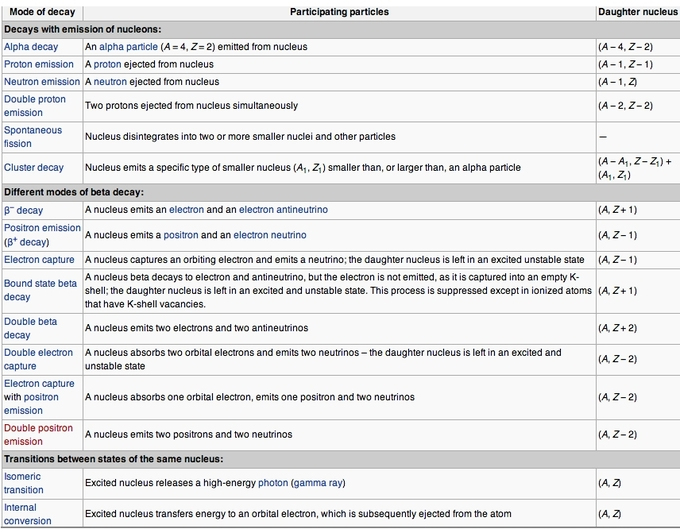#### Learning Objective

• Compare the relative penetrating power of the three types of nuclear radiation

#### Key Points

• The radioactive decay products we will discuss here are alpha, beta, and gamma, ordered by their ability to penetrate matter. Alpha denotes the largest particle, and it penetrates the least.
• Alpha particles carry a positive charge, beta particles carry a negative charge, and gamma rays are neutral.
• An alpha particle is made up of two protons and two neutrons bound together.
• Beta particles are high energy electrons.
• Gamma rays are waves of electromagnetic energy, or photons.

#### Terms

• alpha particleA particle consisting of two protons and two neutrons bound together, identical to a helium nucleus.
• beta particleA high energy electron released during beta decay.
• gamma rayHigh-energy wave of electromagnetic energy.
• isotopeA variant of a particular chemical element, which shares the same number of protons as other atoms of the element, but differs in its number of neutrons.

Radioactive decay occurs when an unstable atomic nucleus loses energy by emitting energy in the form of emitted particles or electromagnetic waves, called radiation. Isotopes are atoms of the same element (thereby having the same number of protons) which differ in the number of neutrons in their nucleus. Some isotopes of a given element are more unstable than others, causing a nuclear reaction which releases energy to achieve a more stable nuclear configuration. Such isotopes are radioactive, and are referred to as “radioisotopes.”

## Types of Decay

There are many types of emmitted particles and radiation that radioisotopes produce when they decay. The types we will discuss here are: alpha, beta, and gamma (listed in increasing ability to penetrate matter). Alpha decay is seen only in heavier elements greater than atomic number 52, tellurium. The other two types of decay are seen in all of the elements.Table of modes of radioactive decayRadionuclides can undergo a number of different reactions, summarized here. A nucleus with mass number A and atomic number Z is represented as (A, Z). The column “Daughter nucleus” indicates the difference between the new nucleus and the original nucleus. Thus, (A − 1, Z) means that the mass number is one less than before, but the atomic number didn’t change.

## Alpha, Beta, Gamma Composition

Alpha particles carry a positive charge, beta particles carry a negative charge, and gamma rays are neutral. Alpha particles have greater mass than beta particles. By passing alpha particles through a very thin glass window and trapping them in a discharge tube, researchers found that alpha particles are equivalent to helium (He) nuclei. Other experiments showed the similarity between classical beta radiation and cathode rays; they are both streams of electrons. Likewise, gamma radiation and X-rays were found to be similar high-energy electromagnetic radiation.

The three types of radiation have different levels of penetrating power. Penetrating power refers to the energy with which the radiation particles are ejected from the atom. The higher the energy, the more the particles or light produced by radioactive decay will penetrate a substance.Penetration of the modes of decayAlpha particles can be completely stopped by a sheet of paper. Beta particles can be stopped by aluminum shielding. Gamma rays can only be reduced by much more substantial mass, such as a very thick layer of lead.

## Alpha Decay

An alpha particle (α\alpha) is made up of two protons and two neutrons bound together. This type of radiation has a positive charge (due to the presence of two protons). An alpha particle is sometimes represented using the chemical symbol He2+, because it has the same structure as a helium atom missing its two electrons—hence the overall charge of +2. Their massive size (compared to beta particles, for instance) means alpha particles have very low penetration power. Penetration power describes how easily the particles can pass through another material. Since alpha particles have a low penetration power, the outside layer of the human skin, for example, can block these particles.

Alpha decay occurs because the nucleus of a radioisotope has too many protons. A nucleus with too many protons causes repulsion between these like charges. To reduce this repulsion, the nucleus emits an α particle. Examples of this can be seen in the decay of americium (Am) to neptunium (Np).

## Beta Decay

In radioactive nuclei with too many neutrons, a neutron can be converted into an electron, called beta particle. Beta particles (β) have a higher penetration power than alpha particles (they are able to pass through thicker materials such as paper).

During beta decay, the number of neutrons in the atom decreases by one, and the number of protons increases by one. Effectively, a neutron was converted into a proton in the decaying nucleus, in the process releasing a beta particle. Since the number of protons before and after the decay is diﬀerent, the atom has changed into a diﬀerent element.

## Gamma Decay

Some decay reactions release energy in the form of electromagnetic waves called gamma rays. Gamma radiation (γ) is part of the electromagnetic spectrum, just like visible light. However, unlike visible light, humans cannot see gamma rays, because they have a much higher frequency and energy than visible light. Gamma radiation has no mass or charge. This type of radiation is able to penetrate most common substances, including metals. The only substances that can absorb this radiation are thick lead and concrete.

Gamma decay reactions occur if the energy of the radioisotope’s nucleus is too high, and the resulting atomic number and atomic mass remain unchanged during the course of the reaction.